# Synthesis by Frequency Analysis

## introduction

We saw on the tutorial, Real-Instrument Synthesis, that there are three fundamental ways to synthesize the sound of real musical instruments: resampling, frequency analysis, and physical modeling. In this tutorial we will learn synthesis by frequency analysis. The backbone of this technique is additive synthesis, that is, adding together simple sinusoidal frequencies to obtain one final sound wave. This is easier said than done, because you need to know exactly which frequencies to use and how strong each component must be.

There is a common computer program for taking a sound waveform and calculating its frequency components: it's called an FFT, which stands for Fast Fourier Transform. You can find the source code for an FFT all over the place. In particular, there is a source listing in the famous book, Numerical Recipes in C. Rather than spend the time typing the source code, just use the FFT that comes part of the Scilab mathematics program. It will be as simple as typing

```    Frequencies  =  fft(waveform, -1);
```

There is one little hitch (we knew this was coming!): you can't just take the frequencies from this program and incorporate them all into a waveform using additive synthesis. Try it. You will get the same waveform back that you entered into the FFT program--exactly the same waveform. It will sound like your sound file looping over and over. That's why this tutorial is necessary, because you have to learn which frequencies, that is, spectral components from the FFT to use. And that means you need to understand how to "read the FFT."

## 2. how to read the fft

The Free Man's fourier theory: In order to understand how to read an FFT you need to completely understand fourier theory. After you know how to read an FFT you will completely understand fourier theory.

For many situations it is enough to know that the FFT tells you what frequencies are in your sound wave. The newby electronic musician may need to consult a simple tutorial on what is fourier theory? by and by. Then, as certain things don't make sense, you might need to consult a tutorial on how to understand fourier theory. If you're ready for college graduate-level mathematics, there is a complete description of this topic at the Center for Computer Research in Music and Acoustics (CCRMA).

#### what is fourier theory?

Fourier Theory is the science of additive synthesis.

1. The Forward Transformation: Take a waveform and find one of its frequencies.

2. The Reverse Transformation: Add all the frequencies to get the waveform back.

The FFT program does both the forward and reverse transformation for you. With the Scilab program you take your sound wave data, say the array called y, and plug it into the FFT function:

```    fy  =  fft(y, -1);
```

which gives you all the frequencies that make up your sound wave. The reverse transformation is done in Scilab with the following command:

```    y  =  fft(fy, 1);
```

which gives you the original waveform back, given the frequencies it contains.

Example 1:

Let's say you have a pure cosine waveform and you want to find its frequencies. Well, there should only be 1 frequency because it is a pure sinusoid. Let's create an arbitrary cosine waveform with Scilab. Make it simple so that it's easy to see on a plot. Let's say the frequency is 1 hz, and let's pretend the sampling rate is only 128 samples per second (WAV files are sampled at 44100 hz). We will make a waveform that has a duration of 1 second for simplicity. Then enter the following Scilab commands:

```    Fs  =  128;             // the sample rate
f   =  1;               // the frequency
N   =  1 : 128;         // an array of numbers counting from 1 to 128
x   =  2 * %pi * (f / Fs) * N;      // the input to the cosine
y   =  cos(x);
```

Recall from the earlier tutorial, creating a sound file, why we must write the cosine argument x with the sampling rate as above. Now the array called y has one complete cycle of a cosine wave. The frequencies can be calculated by putting the cosine wave, y into the FFT function. Enter the following Scilab command:

```    fy   =  fft(y, -1);
```

Now the array called fy contains the frequencies. These are complex numbers, so you have to plot the real part and the imaginary part separately.

```    plot2d3(x,  real(fy))
```

which will look like the following plot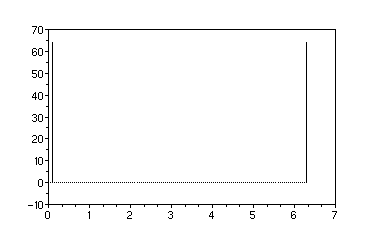In the above plot of the FFT of cos(x) there are two vertical bars, one at the beginning and one at the end. These are the frequencies. There is really only one frequency: the one at the beginning. The frequency bar at the end is its mirror image, and this will be explained below.

The above plot is just the real part of the complex number array. To plot the imaginary part of the array we would use the same command as above but replace real(fy) with imag(fy). Since this was a cos(x) function there is no imaginary part. If we had used sin(x) for our test waveform instead of cos(x) there would only be imaginary numbers, and the real part of the array would be all zeros. Be forewarned that the "zero" elements of this array will usually not be exactly zero. The FFT calculation always results in some numerical noise. This is especially important when comparing the real components to the imaginary components. If we examine the numbers in the fy array we will be surprised to find that numbers that should be zero are very small, but not zero. For example, look at the frequency element for the above plot, which is fy(2). Just type fy(2) at the Scilab command line. We get the following result:

```    ans  =

63.922909 + 3.1403312i
```

The imaginary part of this spectrum is theoretically all zeros, but this FFT calculation came out with 3.1403312 for the imaginary part of this frequency. That is numerical noise. You will need to watch for this as you apply your frequency analysis to music synthesis.

#### what do the fft data points mean?

However many data points there were in the y array, there will be the same number of data elements in the answer, fy. What do the data elements in fy mean? Each data element will be a complex number. Its absolute value is the magnitude of the sinusoid, corresponding to the frequency at that element, that helped to make the original sound wave.

The data values are complex numbers, and this will be explained below. The real part of the complex number is a cosine wave and the imaginary part is the negative of a sine wave.

#### what are the frequencies in the fft?

The first data element, fy(1), is not a frequency: it is the "DC" offset of the waveform, which means that if the waveform is not evenly centered in the vertical direction adding or subtracting this number will align it. The first actual frequency, fy(2), is found by calculating the reciprocal of the time span of the original sound wave. So if the length of the sound sample was 0.1 seconds, the first frequency component will be 10 hz. Then the second frequency component will represent 20 hz, and the third will represent 30 hz, and so on.

So the number in fy(2) is the magnitude of the sinusoid whose frequency is the reciprocal of the time span. Each frequency after the first frequency is an integer multiple of the first, i.e., 3, 4, 5, 6, 7, ..., (N/2)-1 where N is the number of data points in the sound wave file.

In the above plot we graphed the spectrum using the angular frequency variable x. Notice that the horizontal range of the graph runs from 0 to 2 pi. In digital signal processing frequenies are often plotted like this on an axis from -pi to +pi. But it would be easier to see the frequency values if we use a different variable for the horizontal axis. If we multiply the x variable by a factor of the sample rate over 2 pi the axis will be displayed in units of frequency:

```    q   =  x  *  (Fs / (2 * %pi))  -  f;
```

or, the same thing would be

```    q   =  f  *  (N-1);
```

We have to use an array that counts from 0 to N-1 for it to come out right because the first frequency is actually in the second array value. Now replot the spectrum using this new variable to show the frequencies (and zoom the plot to the beginning):

```    plot2d3(q,  real(fy))
```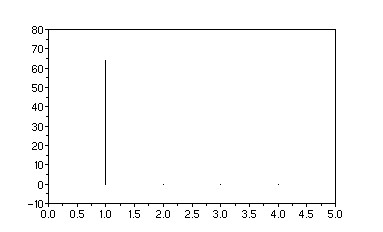Now the above plot shows the true frequency values on the horizontal axis.

#### only half of the fft data is needed?

Say there were 100 data points in the original sound wave. There will be 100 data values in the FFT that you calculate, and only the first 50 of them are uniques values. The other half of the data values are the reflection of the first half. Values 51 through 100 will be exactly the same as the first half except for two things: (1) they are in reverse order, and (2) the imaginary parts are opposite in sign.

The second half of the FFT is commonly called the negative half, and people who work with this every day typically move the second half of data to the beginning before they print out a plot. Calling it the negative half implies that there are negative frequencies. This will make sense if you represent sinusoids with an exponential function, which is a deeper subject we can discuss later.

You can move the reflected spectrum from the upper part of the array with a built-in Scilab command.

```    fftshift(fy)
```

So, to display the spectrum in the "canonical" form, that is, from -pi to +pi and show just the array index numbers on the axis,

```    plot2d3(N, fftshift(real(fy)))
```

which looks like this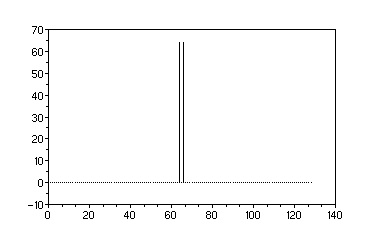You would normally also shift the index numbers so that 0 would also be in the center of the plot, which would put the right-hand frequency at index +1 and the left-hand frequency at index -1. The above plot shows how the reflected spectrum was shifted. If we zoom to the center of the plot we can see the new index numbers: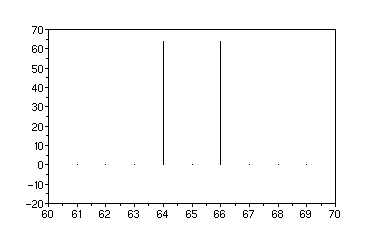The reflected frequency in now at index 64. The shift is equivalent to a cut-and-paste of the second half of the array to the beginning. Of the total N array elements, the second (N/2) elements are inserted at the beginning, and now the DC component is at the (N/2)+1 position, and the first frequency is now at the (N/2)+2 position.

You don't need the reflected spectrum for your additive synthesis--it is an artifact of the mathematics. You may as well just plot the first (N/2) data points from the FFT.

#### why are the frequency values complex numbers?

The frequency values are comples numbers, which means each one is a set of two values: the "real" part and the "imaginary" part. The bottom line is that the imaginary part represents the phase of the sinusoid. We have seen that any waveform can be constructed from a set of sinusoids, but we never said that the sinusoids all needed to start from 0. In fact, this is why I have used the word sinusoid up to now instead of sine and cosine. The sine and cosine are both just sinusoids that are shifted relative to each other by 90 degrees in phase. To be more specific, phase means the phase angle argument to a sine or cosine function.

A complex number is kind-of like a vector. You can draw a graph, and instead of calling the vertical axis the "y" axis, call it the "imaginary" axis and write an "i" (mathematicians use i, engineers use j) next to it. If you mark a point on the horizontal axis with the real part of the number and mark a point on the vertical axis with the imaginary part of the number, you can draw a rectangle whose height is the imaginary number and whose width is the real number. Now if you draw a diagonal line from the origin of the axes to the corner of the rectangle you will have a vector called the phasor. For sinusoids the phasor is a vector whose tip always lies on a circle of radius 1, and the phase is the angle this phasor makes with respect to the horizontal axis as it turns around the circle.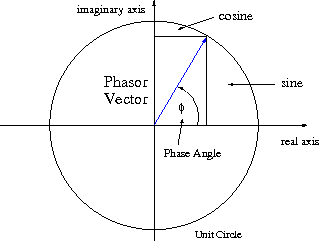The unit circle above defines the sine and cosine. The sine is the distance above the horizontal axis, and the cosine is the distance from the vertical axis. Imagine that the phasor arrow is turning around the circle in the counter-clockwise direction. One complete revolution corresponds to one period of a sinusoidal waveform. The phase angle is the same as the variable x we used above, and that is why its range is from 0 to 2 PI, or -PI to +PI. The phasor is meaningless unless we show both its length and the angle it makes to the axes. These two pieces of information can be represented equivalently by the distance from each axis that the tip of the arrow makes, as can be seen on the graph.

It takes 2 numbers to represent the value of a sinusoid, and you can choose two different systems to express these numbers. Engineers refer to these two systems as rectangular coordinates and polar coordinates. That is, you can represent the two values of a sinusoid as either,

1. Real and Imaginary = rectangular coodinates
2. Magnitude and Phase = polar coordinates

In practical terms, you need both a sine and a cosine to perform your additive synthesis project. The real and imaginary values from the FFT calculation tell you how large each one must be. The real value is the magnitude of the cosine, and the imaginary value is the magnitude of the sine. And you must reverse the sign of the imaginary value before you use it for additive synthesis.

## how to understand the frequencies

Now that you know which data values go to which frequencies we can start understanding them. You will be surprised when you do your FFT calculation with, say Scilab, on a simple sound waveform, and you see that THERE ARE MORE FREQUENCIES THAN YOU THOUGHT. This is because you need to learn how to understand fourier theory.

Be aware that mathematics doesn't necessarily correspond to reality. A "frequency" in fourier theory is a very specific object, and it's not exactly what a musician would call a frequency. In your sound wave file, the amplitutde of the sound doesn't have to be constant over time. The overall amplitude probably moves up and down over the duration of the sound file. In music synthesis we say that the sound has an envelope. Let's say you go to your analog synthesizer and switch the oscillator bank to a pure sine wave. Now record a sound file in which you play a single sine wave note, say Concert A, but gradually turn up the volume as you record. If you now perform an FFT calculation on your sound file you will get more frequencies than just the 440 hz fundamental. I'm not talking about any nonlinearities in your oscillator or even the numerical noise in Scilab's FFT algorithm. You have lots of frequencies because the amplitude was not constant.

A "frequency" in fourier analysis is a sinusoidal variation with time, and the only information it conveys is the time period between its peaks. There is no information about when it started or when it stopped, and there is no information about how loud it is. In advanced mathematics, because of the conventions used in The Calculus, these fourier components have been said to be infinitely long: they extend from minus infinity to plus infinity. But infinity just means that there is information missing--in this case the information about the endpoints of the sinusoidal wave train is missing. But in fourier theory any waveform can be represented by these basic building blocks, these sinusoidal components. So when the original waveform has an envelope shape that changes with time, this envelope information must be included with more "frequencies" in the fourier sense of the word.

The amplitude envelope of the sound waveform is said to be created by adding just the right set of frequency components, all with just the right height and phase shift, so that they either combine or cancel in just the right time to make the envelope. But we have seen that when any sinusoidal wave shapes are combined the result is a waveform that always repeats itself. So, in fourier theory we can create a time-varying envelope by adding more frequencies, but this envelope repeats itself. It is understood that we only consider one of these repetitions in our calculation. Your original waveform obviously doesn't repeat itself. But the frequencies you get from a fourier calculation, such as the FFT, are sufficient to create your wave shape, but which will repeat itself if you let your time endpoints extend in each direction in time.

This property of the fourier calculation, that the envelope created by adding the given set of frequencies repeats itself, is the underlying reason for the subject of "windowing" in digital signal processing. A book on the FFT will almost surely have a chapter on windowing. The reasoning is this: the fact that you have a finite piece of some wave shape implies that you did not necessarily cut it out exactly between its repetition points. But the FFT is a fourier calculation, and so it will find a set of "frequencies" that creates your cut from the waveform and repeats itself. This is not just a property of an FFT or even of a discrete fourier transform. It is a general property of any fourier calculation: the fourier series, the fourier transform. With the analytical forms of the fourier calculation it is assumed that your calculation is performed between endpoints of one complete repetition of the waveform. In fact, it is implicit in the formulas.

So, these extra "frequencies" in the spectrum come from two sources: a time variation, or envelope, of the waveform, and taking a time span that does not correspond to one complete repetition of the waveform. In engineering, the extra frequencies are usually attributed to the circumstance of truncating the waveform: taking a finite piece of what is really indefinitely long. This is another way of saying that the calculation was not performed between the points where the waveform repeats itself.

This is the most basic obstacle to understanding the FFT spectrum: all the time variation of the original waveform is produced by adding "frequencies" that, themselves, have no time variation. Here is a rule of thumb about what frequencies are what in the spectrum (see more):

1. Frequencies that are close to each other create the time envelope.
2. Frequencies that are far apart create the harmonics.

As you will see in how to understand fourier theory, when you multiply frequencies they modulate each other. You could think of the time envelope of your waveform as a slow frequency being multiplied by a faster frequency, each of these having constant amplitudes. There is advanced mathematics to wrestle with this, and its called the Modulation Theorem. What happens when you modulate?. For the sake of clarity, let's call one waveform "the carrier" (the faster one), and let's call the other (slower) waveform "the modulator." Think of the waveform called the modulator as producing an effect on the one called the carrier (it doesn't matter which name we give to which). In the simplest circumstance, where both the modulator and the carrier are simple sinusoids, the modulator splits the carrier into two frequencies that are equally spaced from the original frequency by an amount equal to the modulator's frequency.

As a rule of thumb, you can remember how this works by referring a simple trig identity:

```    cos(u) cos(v)  =  (1/2)[ cos(u - v)  +  cos(u + v) ]
```

## 3. the structure of the musical maveform

The sound wave created from a musical instrument has certain features that we can use to help break it down more easily. Musical instruments are resonators. You may be interested in the physics of resonators at a deeper level, but for our purposes in fourier analysis it is sufficient to understand that the spectrum consists of evenly-spaced frequencies. The sound waveform of a resonantor, in a steady state condition, has an easily recognizable fundamental cycle with easily recognizable harmonics seen as miniture cycles superimposed on the larger cycle. A resonator, that is, an ideal resonator (see more) in the mathematical sense, will have harmonic frequencies that are integer multiples of the fundamental frequency.

```    f1   =  the fundamental frequency

f2   =  f1  *  2

f3   =  f1  *  3

f4   =  f1  *  4

.       .

.       .
```

This property, this ordered structure of the spectrum, makes it a lot easier to distinguish the harmonic content from the envelope. We have seen in our tutorial on how to understand fourier theory that when you modulate a waveform, the spectrum of the original waveform appears "smeared out" in the final waveform.

Here is the shortcut: if your waveform has an envelope that changes no faster than the fundamental period of oscillation, then the spectral smearing of one frequency will not cross over the neighboring frequencies. You can easily see where the locations of the unmodulated harmonics are because there are now groups of clustered frequencies that have their centers evenly-spaced on the frequency graph.

## the envelope frequencies

The first approach to demodulating the waveform is to simply choose individual frequencies at the centers of the spectral groups. Let's look at the saxophone note that we resampled in the previous tutorial, the file called asn5a.dat. The FFT spectrum (the cosine part) of asn5a.dat is plotted below.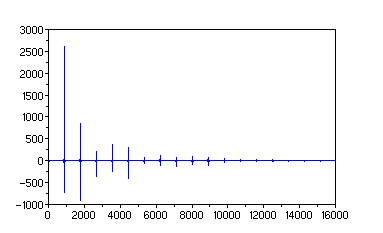The saxophone spectrum has a nice, clear resonator spectrum, as can be seen on the graph above. At this resolution we can't even see the clumps of frequencies: the groups look like single harmonics at evenly-spaced distances across the spectrum. We need to zoom the graph down to one of the harmonics to see the cluster of frequencies. In the graph below the second frequency group is shown.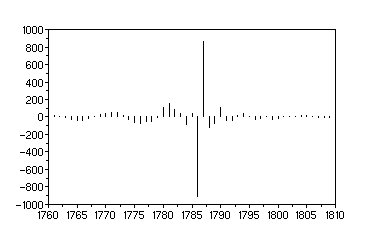The first caveat to note is that not all of these "smeared out" frequencies are due to the envelope. If we simply pick single harmonics at the centers of these clumps we will get a saxophone sound, but it will be a very artificial-sounding saxophone. Musical instruments are not perfect resonators, that is, they are not idea resonators to a mathematician. A musical instrument is more like a resonator that is attached to other, smaller resonators that modify its sound. It is like a system of coupled resonators like the way a molecule is a system of coupled atoms. As we saw in the physics of resonators, coupled resonators modulate each other. The spectrum of a musical instrument will have harmonics that are almost, by not quite, evenly-spaced. It is as if the harmonics are slightly detuned. This "imperfection" (to a mathematician) of the spectrum is what gives the richness and uniqueness to the sound.

The shape of the frequencies in the above graph is similar to a sinc function. This is easily recognizable as the effect of a truncated wave train. A true sinc function is the envelope (in the frequency domain) resulting from pinching off the wave train, leaving lengths of zero data on each end. Your book on digital signal processing will tell you all about it. The subject of windowing was developed to deal with this problem. For our work in music synthesis we will just try to work around it by taking sections of the sound wave that do not contain zero data on each end.

If you start using the windowing techniques in your digital signal processing book you will be going down the wrong path. The book will mention zero-padding. You DO NOT want to pad your data with zeros. In engineering jargon, this will increase the "main lobe" of that sinc function we mentioned.

## the harmonic frequencies

In order to get around the problem of the sinc function on the spectrum, let's just take a section from the sound wave that is as close to constant amplitude as possible. We should make this cut as carefully as possible to minimize spurious frequecies that result from a fourier transform between non-repeating points. In other words, make the beginning of the cut at a location that looks like the beginning of a sinusoid, and make the end of the cut at a location that resembles the end of a sinusoid (recall that we followed this technique in the tutorial on resampling).

Let's take a cut from the saxophone note, the file asn5a.dat, and perform an FFT on it. Take the samples from a suitably constant section, say 31039 to 37708, the section of waveform will look like the following graph.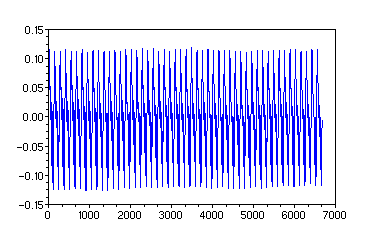The FFT of this small section of the waveform (the imaginary part) is plotted below. Note that the imaginary part of this FFT is much larger than the real part. The location in the fundamental waveform cycle (the phase) where we start the cut makes a difference.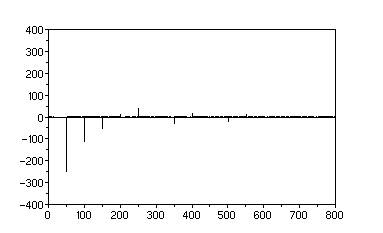If we zoom the graph down to the beginning of the spectrum we see something very telling. The fundamental frequency is expanded to fill the whole plot.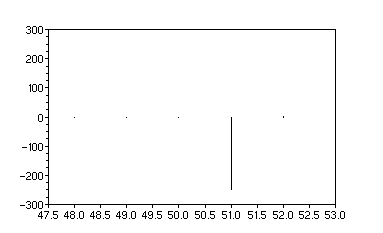There is no "smearing" of the frequency! In fact, if you zoom on any of the frequencies in the imaginary part of the spectrum you find the same circumstance. The real part of the spectrum is about ten times smaller in amplitude. This cut of the waveform is very close to being aligned with respect to the phases of its harmonics. A part of the real part of the spectrum is shown below.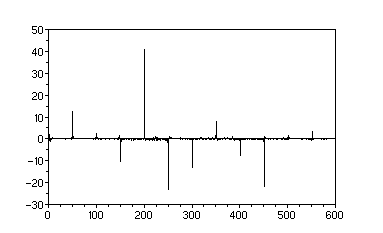With this FFT algorithm, the one in the Scilab software, I am suspicious of any frequencies smaller in magnitude than about 5 percent of the maximum. This is because the algorithm is very prone to numerical noise. In this case, I would look carefully at anything with a magnitude less than 10. A zoom of the fourth frequency in the real spectrum is shown below.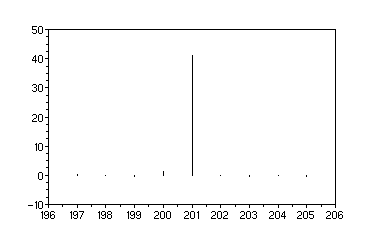Again, there is virtually no smearing of the frequency. In effect, the structure of this waveform is very simple. It is clear that the harmonics all have different phases with respect to each other, and if we were to use this spectrum for our additive synthesis "real instrument" synthesis, we should respect these phases. We want to enchant the ear, not go home early.

## 4. time stretching a short section

While we have a nice, little cut from the sound wave, this would be a good time to show how a sound is time-stretched with an FFT. That is, we would like to make the sound have a longer duration but without changing the pitch.

In principle, time-stretching the sound wave requires first removing the envelope, then "oversampling" (adding more samples) the constant amplitude harmonics that are left over, then reapplying an envelope. But it will often happen that the harmonics also have their own envelopes--the whole sound evolves with time. Therefore, with the most interesting sounds there will be many steps in the work, and each individual sound will require its own approach.

For this simple example a small, fairly uniform section of the sound has been chosen so that demodulating the envelope(s) is circumvented. This leaves only the task of adding more samples to make the sound longer. The astute reader should reflect that this is the frequency domain counterpart to looping, that is, we are merely adding more samples but in the frequency domain instead of the time domain. We will use the following principles to accomplish this goal:

1. The same number of samples exist in both the time domain and the frequency domain
2. The total time duration of the sound is the reciprocal of the frequency spacing of the spectrum

We will add more samples in the frequency domain so that the resulting sound wave in the time domain will have a longer duration. But we must keep exactly the same number of non-zero frequencies and increase their frequency spacing with respect to each other. Shifting the non-zero frequency set into the new frequency locations must be done very carefully. If any one of the new frequencies is misplaced the method fails.

The following example can be reproduced with the Scilab script file fft3.sci. In it, we use the saxophone note data file, asn5a.dat, that we created in the resampling tutorial. Read the entire saxophone note data into Scilab with the following commands,

```    Fs = 44100;
tx = A(:,1);
y = A(:,2);
```

Now check the data by plotting the waveform with

```    plot(tx, y)
```

We may want to plot this data verses frequency number instead of time, so that the following commands will be useful,

```    m = max(size(tx));
tn = 1:m;
plot(tn, y)
```

In order to obtain the small section that was shown above, the following Scilab code should be run. The sample points between 31039 and 37708 were identified as a fairly constant amplitude section:

```    n1 = 31039;
n2 = 37708;
q1 = n1:n2;          // array indices of the cut
y1 = y(q1);          // y data from the cut
tx1 = tx(q1);        // time of the cut
m1 = max(size(q1));  // size, in samples,  of the cut
```

Now plot this short section of the waveform

```    tn1 = 1:m1;
plot(tn1, y1)
```

And now the spectrum of this small cut is obtained by performing the FFT on the y data as follows

```    fy1 = fft(y1, -1);  // FFT of the cut data
```

You can calculate the frequency separation between frequency samples with the following line, where Fs is the common sample rate of 44100,

```    ff1 = Fs / m1;
```

Here is a trick for cleaning up the noise in the spectrum by finding any frequency values that are less than some threshold. The value of this theshold was obtained by trial-and-error.

```    ns = find(abs(fy1) < 5);    // indices of the noise
zz1 = zeros(fy1);           // array of zeros
fyy1 = fy1;                 // copy the frequencies
fyy1(ns) = zz1(ns);         // zero-out only the noise
fyy1(1) = 0 + %i * 0;       // zero out DC
fyy1 = fyy1 * 10;           // increase SNR
```

Now we should create an array to hold ten times as many samples so that we can copy the frequencies from the old spectrum.

```    // size of stretched data
M2 = m1 * 10;
// placeholder for larger spectrum
fyyy1 = zeros(1,M2) + %i * zeros(1,M2);
```

Identify the non-zero frequencies from the old spectrum

```    nq = find(abs(fyy1) > 0);       // indices of the nonzero data
snq = max(size(nq));            // how many of these indices are there
snq2 = int(snq/2);              // half of that size (half spectrum)
```

The following Scilab code lines perform the relocation of the frequencies into the new, larger spectrum. This is a critical part, because one misplaced frequency will ruin the whole project. If you consider the first half of the old frequency spectrum, it is obvious that the frequency spacing must be increased by the same factor as the increased sample size, in this case 10. But the spectrum in the FFT data comes to us offset by one index because of the DC component at the beginning. We need to find the index values for the frequencies as if the DC component was not there. The following code line is used to obtain that result,

```    nq2 = nq(1:snq2) - 1;
```

Now we can multiply these index values (for the first half of the spectrum) by the time stretch factor so that there spacing will be correct. After that we will need to add 1 again in order to restore the presence of the DC component.

```    nqq = (nq2 * 10)+1;     // multiply the factor and shift DC back
```

This new set of array indices, nqq, is the set of indices in the new array where the first half of the spectrum will be placed. We will now use a loop to count through these indices and copy the non-zero frequencies from the old spectrum into the new spectrum. The frequencies in the upper half of the spectrum are the mirror image of the lower half, so we must count backwards from the end so as to copy these in reverse-order.

```    for i = 1:snq2,
//
//  first half
//
fyyy1(nqq(i))  =  fyy1(nq(i));
//
//  2nd half (mirror image of first half)
//
re1 = real(fyy1(nq(i)));
im1 = imag(fyy1(nq(i)));
fyyy1(M2 - nqq(i) + 2)  =  re1 - %i * im1;
end
```

The placement of the upper-half frequencies will depend critically on the offset used (in the above code a value of 2 was used). Pay attention to the fact that the imaginary values that were copied from the lower half to the upper half were reversed in sign. You would, of course, not reverse the signs if you instead copy the original upper-half frequencies into the new spectrum.

Now we "fake out" the FFT program by putting this new spectrum in place of the old spectrum and calculate the inverse FFT, obtaining a time-domain waveform.

```    yyy1 = fft(fyyy1, 1);
```

The FFT program will give you back complex values for the data samples. The imaginary parts are ignored, so we pick out the real values from the data to be our new sound waveform,

```    yyy1 = real(yyy1);
```

If you plot this new data, you should see a waveform with (practically) constant amplitude:

```    plot(yyy1)
```

Now export the data into a WAV file and listen to the result,

```    wavwrite(yyy1, Fs, 'asn5a_stretch.wav');
```

Compare, if you will, this WAV file, asn5a_stretch.wav with the WAV file of the original saxophone note asn5a.wav. The resulting time-stretched sound is a little artificial, which may be attributed to some degree to the fact that we "cleaned up" the spectrum, but mostly due to the circumstance that this was a short piece "taken out of context" from a sound whose time variations are part of its identifying features.

It should occur to the clever programmer of visual, 3D rendering that the above procedure could be used to create, or grow, a texture from a smaller sampling and a two-dimensional FFT program.

The small cut from the total sound waveform is the starting point for synthesizing complete instrument notes. We can take this in either the time domain or the frequency domain. In the time domain, we can create stretched-out samples and assemble a sound font for resampling. In the frequency domain, we can take the frequency amplitudes and perform additive synthesis with a program such as Csound--we still need separate frequency bands corresponding to each instant in the life of the note, just as we need several sound samples to assemble a sound font in the time domain. Which method to use will depend on which is easier, which will depend on how many frequencies there are.

## 5. analyzing the time evolution

The musical note is obviously not one unchanging entity, like the time-stretched file we created above. There is a beginning, an end, and many stages in between, depending on the instrument. Moreover, these various stages in the life of a note are not just amplitude changes. It takes more than attack, decay, sustain, release (ADSR) to synthesize these stages. The frequencies are also changing during the life of the note.

The time evolution of the saxophone note we have been using is shown below with some arbitrary time intervals indicated.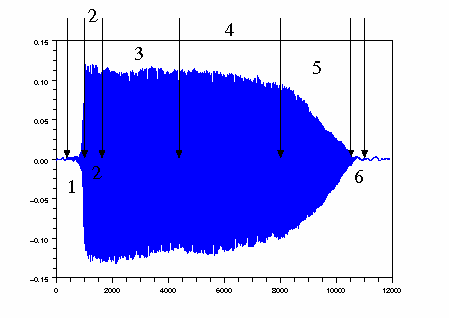The real-sounding synthesis of this note will need at least this number of separate frequency sets (using the frequency method) or sound samples (using the resampling method).

## the attack

The intervals 1 and 2 will comprise the attack of the note. The tiny waveform seen in interval 1 may not seem important, but this determines the sharpness and texture of the attack. Human auditory pattern recognition is surprising in some respects. The least little differences in sound patterns, that a typical engineer would consider insignificant , can be heard by the human ear. The average listener can distinguish a time lag between two notes with as little as 2/100 of a second difference. This little time interval between 1 and 2 on the above graph will make the difference between a gourmet saxophone note and a drive-through saxophone note.

The attack will usually contain a lot of high frequencies, and for this reason an attack interval simulated by the frequency method might require too much computation--it might make more sense to just resample the attack part of the note. If you have hundreds of notes in your score the difference might be hours instead of minutes in computer computation.

The waveform in interval 1 is show below. The waveform is fluctuating widely. This is the beginning transient action of the sound before resonations have stabilized.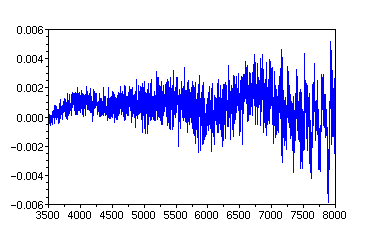If you look hard in a zoomed section you'll see that the fundamental oscillation has not emerged yet (see below). The sound is still deciding what kind of motion it will become. It is the physics of transient motion that the wavelengths which fit between the walls take some time to build up strength, while all the others take some time to destructively interfere and add to zero.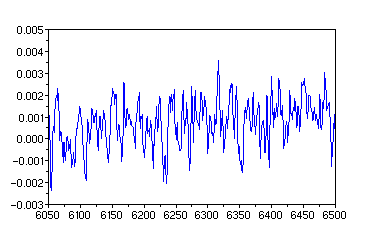The FFT frequencies for this time interval are shown in the plot below.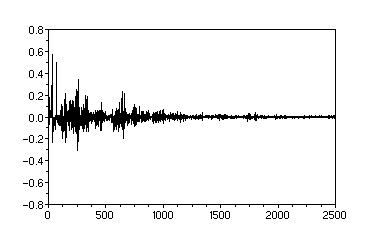There is a big difference between this spectrum and the one we obtained previously for the constant amplitude section. In fact, the computational effort required to reproduce this is enormous. There are "legitimate" high frequencies in this spectrum due to the hiss associated with the start of a woodwind instrument, and there are the "smeared out" fourier frequency artifacts due to the modulation produced by the varying and growing amplitude.

From the point-of-view of computational work, it would be appropriate to resample this part of the waveform.

The latter part of section 1 comprises the bulk of the attack. This is an interesting waveform, plotted below between points that were specially selected.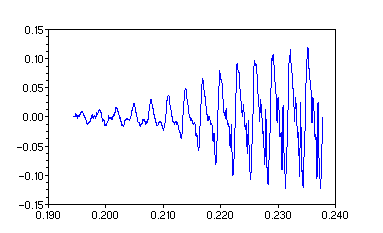The data was chosen

• Where the fundamental mode of the oscillation begins to emerge as the dominant cycle in the waveform.
• Where the waveform just reaches its maximum at the end.
• Beginning and ending at points which imply the same phase shift for a sine wave.

The FFT for this section is plotted below.

The real part: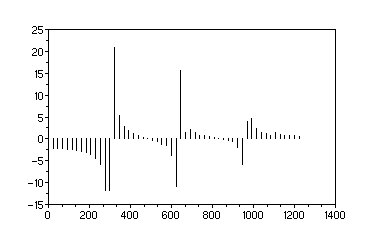And the imaginary part: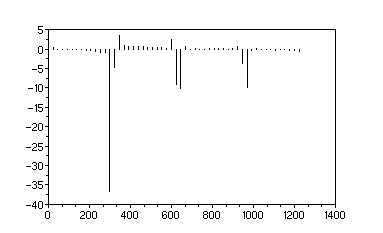We simplified the spectrum when we chose points matching the phase shift at each end. But the spectrum is still "smeared out." Instead of single frequency bars at the resonance lines (integer multiples of the fundamental) there are frequecies in groups around each resonance. These are the result of the increasing amplitude--the envelope. These are the amplitude modulation frequencies.

In additive synthesis you could use this spectrum is either of two ways:

1. Keep the modulation frequencies, and let the envelope arise as a natural consequence of the modulation.
2. Extract only the resonance frequencies, and create the attack manually.

There will be fewer computations and more direct control over the synthesis by using the second method. With the first method, if you don't program its duration correctly the envelope will begin to repeat itself.

In order to perform the second method, that is, remove the envelope, you need to calculate the spectrum successively with time and track the change in the frequency spectrum. If we try to cut out individual waveform cycles and calculate the frequency spectrum on each cycle we will find a time-changing track of each frequency from start to finish. Twelve of the cycles in the waveform above are plotted individually, from top-left to bottom-right, to illustrate the successive cycles.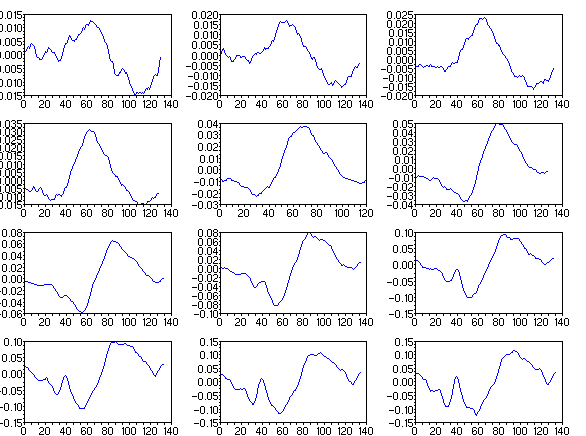There is often some subjective judgement as to where a complete cycle begins and ends. The above individual data arrays form a contiguous set that can be concatenated back to the original waveform.

The first 10 frequencies from each of these FFT calculations is plotted below over the 12 cycles of the waveform.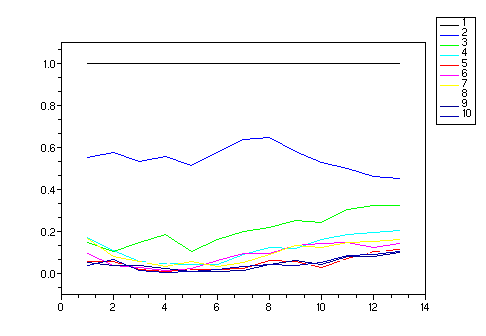The fundamental frequency is shown as 1 throughout the waveform because it is the basis for comparison with the harmonics. With each successive cycle of the waveform you can see what relative amplitude each of the harmonics contributes to the waveform. Bare in mind that each of these FFT spectra contains a small amount of error because the amplitude of each cycle was increasing during its cycle. This small ramp on the amplitude during one cycle will cause a spurious smearing, but there is no place for the smearing frequencies to go except into the resonance frequencies. This could be the time when you try to apply a demodulation algorithm. But it will be a lot less work to apply an (estimated) inverse ramp and then perform the FFT.

This illustrates the additive synthesis method for reproducing the harmonic content of the attack of the waveform. These frequency tracks, as shown above, form the time-varying amplitudes of the sine and cosine oscillators during the attack. The time span of this attack is roughly 0.04 seconds, so that means in your music software (such as Csound) the frequency values must change amplitude at least every 100th sample and preferable every tenth. This is all in addition to the ramp in volume that is the typical attack parameter for synthesizers.

## the sustain

The middle part of this saxophone note is shown as section 2, 3 and 4 above. This note is relatively simple compared to, say, a piano note. Although there will not be much variation in the spectrum between these sections, there is still frequency activity that you want to capture. If you recall the sound of the time-stretched note, it was relatively "unalive". You will need to make successive frequency calculations throught the time interval and employ a frequency time track to your sine and cosine oscillators.

Taking an FFT of a short section at the beginning of the sustain, say, from 0.24 seconds to 0.54 seconds (roughly section 2 on the diagram), we have the following plots:for the time-domain waveform, and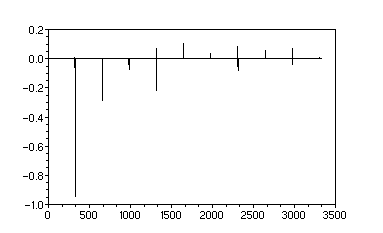for the imaginary part of the spectrum.

Let's compare the first three resonance groups of frequencies here to those found during the rising attack.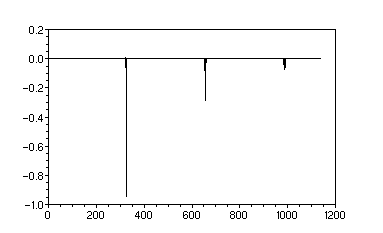It can be seen that there is a similar pattern to the frequencies, but there is much less modulation--the energy of spectrum is not smeared out into the neighboring frequency locations like it was in the plot above for the attack. In fact, if we look at the first group around the fundamental frequency, there are three other frequencies next to the fundamental that are producing a faint "beat".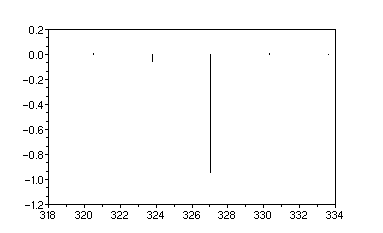This is to illustrate that the closer to a uniform, constant amplitude waveform we have, the closer we get to a textbook resonance spectrum that has only frequencies at whole number multiplies of the fundamental.

## the decay

The decay has a varying amplitude, and so we expect the spectrum to be very smeared-out like with the attack by with different phase relationships.

## 6. assembling frequency tracks

We have seen how the spectrum, not just the amplitude, of this note varies over the life of the note. In order to use additive synthesis to replicate this instrument for this particular note we need a set of oscillators (sine and cosine functions) that change in amplitude throughout the note, and this is not including the overall amplitude envelope.

Care must be taken in selecting the time intervals from which we obtain the spectral amplitudes (the strengths of the sines and cosines) according to the following rules:

1. The time intervals should all form a contiguous set. That is, one starts where the last ended.
2. The size of the time interval will have to be adjusted by trial and error so as to not be too large--the larger the section the more frequency sidebands in the spectrum.
3. This set of frequency tracks forms just one set in a larger "model" of the instrument. In other words, you will need recordings from this instrument that represent various musical expression levels.

To illustrate the time track, the following plot contains the (thresholded) imaginary frequencies during the sustain interval from sample number 10487 to sample number 77984.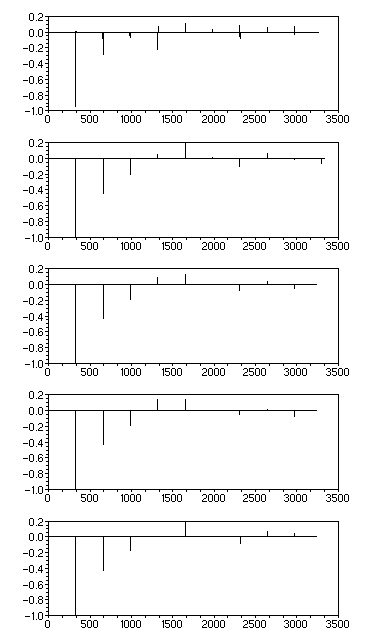Assembling frequency tracks can be automated to some extent, but complete automation is probably wishful thinking. There are too many problems to consider along the way to rely on a one-stroke soultion. Alas, we can't just "point and click" our way to greatness. You're doing brain surgery here. If the subject can get up walk after you're done, then open your front door and shout, "It's alive!"

You may try using this Scilab script, ffttrack.sci, as the first step in creating the frequency tracks. To run this you will also need the Scilab function script, fit_the_track.sci.

You break down your waveform into contiguous subsections by specifying the data sample numbers (counting from 1) in the sound data where to break the sections. The end point of one section is the beginning point of the next. For example, if there are only two sections the sample file will contain just three numbers. Make this into a single-column ascii data file called, say, "sampletrack.dat."

The output from this Scilab script will be a data file called "freqtrack.dat" which will contain the spectrum for each time subsection as a row in the file. The first column will be the time at which this spectrum occurs. The subsequent columns will be the frequency (in hertz), the real amplitude, and the imaginary amplitude repeated in these sets of three (interleaved). The frequency tracks are obtained by assembling individual arrays for each harmonic component by taking the data along the columns. For example, the frequncies for the fundamental, through the duration of the note, are given by the second column in the file. The real amplitudes are given by the third column, and the imaginary amplitudes are given by the fourth column.

Be aware that the spectral components for each time subsection may not, and usually will not, be aligned column-wise in the file unless you choose a particular set of boundary samples with a particular choice of threshold. If the time subsections are short enough, there will be no modulation side bands, only the resonance frequencies, and the components will all line up column-wise. If not, you will need to edit the file by hand and make the frequency components line up by removing sideband frequencies. This is the main issue with this contruction. It will take a lot of programming to make this step automated.

The next step will be to interpolate between this subsection boundary points to create an array of frequencies corresponding to each time sample in the final sound data. You can try this Scilab script, timetrack.sci, to create the interpolated arrays for each frequency component. To use this script, it is assumed that you have run ffttrack.sci immediately before, and its variables are still in the Scilab workspace. After running this script you will have three matrices: a frequency matrix, a real amplitude matrix and an imaginary amplitude matrix. The rows in these matrices correspond to different harmonics in the final sound file. Row 1 is the fundamental frequency, Row 2 is the first harmonic, etc.

The additive synthesis is finally performed by using the Scilab script wavetrack.sci. Each row in the frequency matrix mentioned above is a frequency track, i.e., the time variation os a single resonance frequency throughout the duration of the note.

The work involved with this construction centers around choosing the best sample points at which to subdivide the original sound file. There will be a trade-off between choosing smaller-sized sections and varying the noise threshold. Then the first frequency file, "freqtrack.dat", will still need to be edited to make sure the columns are aligned at similar resonance frequency points. Just search down the frequency columns (2, 5, 8, 11, ...) and the numbers should look close.

## 7. the fft time-stretch method

The frequency tracks method should work fine once the file resulting from the first script is adjusted. But this will entail a lot of hand editing of the data files. As a result of different choices for noise thresholds, some high frequency components may appear and then disappear, only to appear again later. There is significant bookkeeping in the computer code involved. Still, this method may be the right choice for you.

The frequency analysis synthesis may also be performed using the FFT time stretch that was demonstrated above in time stretching a short section. The advantage of the FFT time stretch is that the algorithm doesn't have to keep track of the frequency components. There is a lot less work involved with the FFT stretch method, and it has its own drawbacks.

A very generous person has written a Scilab script for you, time_stretch.sci, which encompasses the end-to-end process of subdividing the original sound file, stretching the sections and reassembling a new sound file. To run this script you will also need the utility script, fft_stretch.sci. The input file for this method is an ascii data file containing one column of numbers, each of which is the sample number at the boundary of a subsection. This is exactly the same kind of input file that is used for the frequency track method defined above. In addition, another input file is used to control how the note will be stretched. A "model" file, an ascii file with two columns, must contain the stretch multipliers in the first column and amplitude boundaries in the second column. There is a noise threshold to be set in the script which determines how many of the small-amplitude frequencies in the FFT's to keep. Start with a value of zero for this parameter and only increase it experimentally by a few percent. You may also create and use an optional threshold file which will let you specify a separate threshold for each subsection, called "thresh.dat".

The most important step for setting up this method is choosing the best subsection boundaries. You may want to oversee this work yourself, as it is too important to be left to the servants. You will need to plot the waveform, zoom the plot down to the resolution where you can see the sample numbers at the zero crossings, and write down those sample numbers. For sound files with a lot of amplitude variation you will need to choose very short time intervals for the subsections.

The second most important consideration for using the FFT stretch method is deciding which intervals to stretch. These are the numbers in the first column of the "model" file. Some intervals are best left unstretched because they lie on sections that vary widely in amplitude or DC level. You should begin the work with all the stretch factors set to 1. Try stretching different sections and listen to the result.

The second column in the "model" file, the amplitudes, should not be used as a method for creating an ADSR envelope--you can do that later. These amplitude numbers should be used for smoothing the resulting data sections.

As an example result from this script, the sound file asn5a.wav, inported as the ASCII file asn5a.dat, was input into the FFT stretch script. A sample number file, sampletrack.dat, was used to subdivide the data into sections. The "model" file, which determines which sections are stretched and the smoothing amplitudes, was modelfile.dat. The stretched sound data can be seen in the following Scilab plot: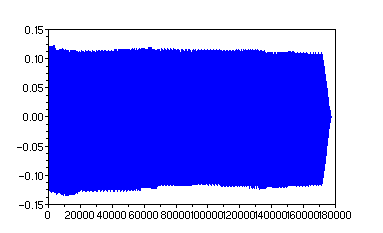After applying a simple ADSR envelope to the data, the resulting sound data is plotted below,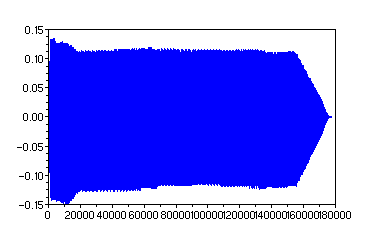The actual sound file, with the envelope applied, is provided for your examination in asn5along.wav. Compare the waveform plots above with the plot of the original wave file shown in the section above, analyzing the time evolution. Compare the apparent bumpiness of the stretched data with that of the original data.

## summary

We have seen how to calculate the frequency spectrum of a sound file by using an FFT program, specifically the one in Scilab. We have seen how to analyse the frequencies in the sound spectrum based on how long the time sample is and whether or not the time sample has amplitude (envelope) variation.

• For a single cycle of a wave form there is no difference between a resonance spectrum and modulation sidebands. Every frequency location in the FFT spectrum may have a nonzero value, and the frequency separation is the reciprocal of the total time of the waveform. The alternative interpretation is that any arbitrary curve shape spanning the data is assumed to be a single cycle in a waveform.
• If the waveform is made to repeat itself (lengthened by adding more copies of the cycle) the frequency lines become separated by more and more zero-valued frequency locations between them. The spectral lines may now be interpreted as resonance lines. Now the frequency separation of the FFT bins are equal to the reciprocal of the time of the whole wave train.
• If the wave train is now given an envelope, the deviation from constant amplitude is equivalent to an amplitude modulation on the waveform. The modulation causes "sidebands" to appear between the resonance lines in the FFT spectrum.
• When a waveform is truncated arbitrarily, leaving zero padding at one or both ends of the sample data, this is just a special case of an amplitude envelope. The sidebands are then known to form a sinc function shape around each of the resonance lines. The more zero padding there is, the more the resonance spectrum become distorted. A typical musical note envelope will produce sidebands roughly resembling the sinc function shape.

We have seen how to extract the harmonic frequencies from a sound file by taking sample sections that are as uniform in amplitude as possible. This may mean trying smaller and smaller time intervals. Sample sections should be taken between the zero crossings of the waveform to minimize spurious frequencies (the equivalent of looping noise in the time domain).

We have seen how a sound file may be stretched, without changing its pitch, by carefully applying fourier frequency analysis and modifying the inverse FFT of a waveform spectrum.

I should mention that the Csound program contains a software tool called PVANAL (phase vocoder analysis) that is perhaps equivalent to the techniques shown here. You should try that technique first before embarking on the work outlined here, as it may prove mor valuable to you.

 Music Synthesis and Physics Home© Alfred Steffens Jr., 2007